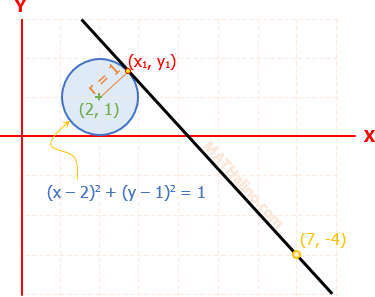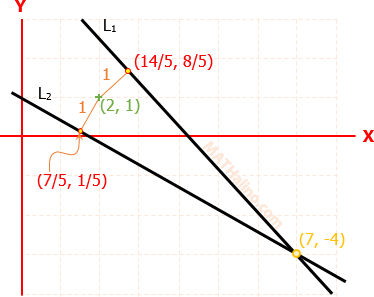# analytic geometry: equation of lines through one point and at a distance from another point

4 posts / 0 new
reyno ramosanalytic geometry: equation of lines through one point and at a distance from another point

can you help me with this problem; find the equations of the lines through (7, -4) passing at a distance 1 from the point (2, 1)

Jhun VertCreate a circle of radius 1 with center at (2, 1). The equation of the circle would be (x - 2)2 + (y - 1)2 = 1. Then draw a line through (7, -4) that is tangent to your circle, you can see that you can draw two such lines, one is above the (2, 1) and the other is below the point (2, 1). You only need to draw one of the lines. Call the point of tangency as (x1, y1) and our task now is to locate this point of tangency. You can find (x1, y1) knowing that this point is common to the circle and the line. Once you get (x1, y1), you have now two points on the line to be used to find its equation.

Jhun VertHere is the details of the solution:$(x - 2)^2 + (y - 1)^2 = 1^2$

$(x^2 - 4x + 4) + (y^2 - 2y + 1) = 1$

$x^2 + y^2 - 4x - 2y + 4 = 0$

The circle above will pass through (x1, y1)
${x_1}^2 + {y_1}^2 - 4x_1 - 2y_1 + 4 = 0$   ←   Equation (1)

Equation of tangent to x2 + y2 - 4x - 2y + 4 = 0
$xx_1 + yy_1 - 4\left( \dfrac{x + x_1}{2} \right) - 2\left( \dfrac{y + y_1}{2} \right) + 4 = 0$

$xx_1 + yy_1 - 2(x + x_1) - (y + y_1) + 4 = 0$

At point (7, -4)
$7x_1 - 4y_1 - 2(7 + x_1) - (-4 + y_1) + 4 = 0$

$7x_1 - 4y_1 - 14 - 2x_1 + 4 - y_1 + 4 = 0$

$5x_1 - 5y_1 - 6 = 0$

$y_1 = x_1 - \frac{6}{5}$   ←   Equation (2)

Substitute y1 = x1 - 6/5 of Equation (2) to Equation (1)
${x_1}^2 + (x_1 - \frac{6}{5})^2 - 4x_1 - 2(x_1 - \frac{6}{5}) + 4 = 0$

${x_1}^2 + ({x_1}^2 - \frac{12}{5}x_1 + \frac{36}{25}) - 4x_1 - 2x_1 + \frac{12}{5} + 4 = 0$

$2{x_1}^2 - \frac{42}{5}x_1 + \frac{196}{25} = 0$

$x_1 = \frac{14}{5} ~ \text{and} ~ \frac{7}{5}$

From Equation (2)
$y_1 = \frac{8}{5} ~ \text{and} ~ \frac{1}{5}$$m = \dfrac{y_2 - y_1}{x_2 - x_1}$

$y - y_1 = m(x - x_1)$

For line L1

$m = \dfrac{-4 - \frac{8}{5}}{7 - \frac{14}{5}} = -\dfrac{4}{3}$

$y - \frac{8}{5} = -\frac{4}{3}(x - \frac{14}{5})$

$15y - 24 = -20(x - \frac{14}{5})$

$15y - 24 = -20x + 56$

$20x + 15y - 80 = 0$

$4x + 3y - 16 = 0$           answer

For line L2

$m = \dfrac{-4 - \frac{1}{5}}{7 - \frac{7}{5}} = -\dfrac{3}{4}$

$y - \frac{1}{5} = -\frac{3}{4}(x - \frac{7}{5})$

$20y - 4 = -15(x - \frac{7}{5})$

$20y - 4 = -15x + 21$

$15x + 20y - 25 = 0$

$3x + 4y - 5 = 0$           answer

Manoj KarmakarI am happy to register this.

• Mathematics inside the configured delimiters is rendered by MathJax. The default math delimiters are $$...$$ and $...$ for displayed mathematics, and $...$ and $...$ for in-line mathematics.